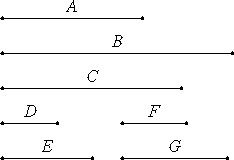# Proposition 20

If one mean proportional number falls between two numbers, then the numbers are similar plane numbers.

Let one mean proportional number C fall between the two numbers A and B.

I say that A and B are similar plane numbers.

Take D and E, the least numbers of those which have the same ratio with A and C. Then D measures A the same number of times that E measures C.Let there be as many units in F as times that D measures A. Then F multiplied by D makes A, so that A is plane, and D and F are its sides.

VII.20

Again, since D and E are the least of the numbers which have the same ratio with C and B, therefore D measures C the same number of times that E measures B.

Let there be as many units in G as times that E measures B. Then E measures B according to the units in G. Therefore G multiplied by E makes B.

Therefore B is plane, and E and G are its sides. Therefore A and B are plane numbers.

I say next that they are also similar.

VII.17

Since F multiplied by D makes A, and multiplied by E makes C, therefore D is to E as A is to C, that is, C to B.

Again, since E multiplied by F and G makes C and B respectively, therefore F is to G as C is to B. But C is to B as D is to E, therefore D is to E as F is to G. And alternately D is to F as E is to G.

Therefore A and B are similar plane numbers, for their sides are proportional.

Therefore, if one mean proportional number falls between two numbers, then the numbers are similar plane numbers.

Q.E.D.

## Guide

This is a partial converse of VIII.18. It says that if two numbers have a mean proportional, then they can be viewed as two similar plane numbers.

#### An example

An example might clarify the details. The variables refer to the outline of the proof below. The numbers a = 18 and b = 50 have a mean proportional c = 30. We’ll see a and b as the sides of the plane numbers, 3 by 6 and 5 by 10, as follows.

When a : c is converted to lowest terms, the result is d : e = 3 : 5. Then f, which is a/d, equals 6, and the number a = 18 is seen as the plane number d = 3 by f = 6. Also g, which is d/c, equals 10, and the number b = 50 is seen as the plane number e = 5 by g = 10. The sides of these plane numbers, 3 by 6 and 5 by 10, are proportional.

#### Outline of the proof

Suppose two numbers a and b have a mean proportional c. Reduce the ratio a : c to lowest terms d : e. Then d divides a the same number of times e divides c; call that number f. Then a is a plane number with sides d and f.

Now since, c : b is the same ratio as a : c, it also reduces to the ratio d : e in lowest terms. Therefore, d divides c the same number of times that e divides b; call that number g. Then b is a plane number with sides e and g.

Furthermore, the two plane numbers a and b are similar since we can show their sides are proportional as follows. From the three proportions d : e = a : c (which follows from a = fd and c = fe), a : c = c : b (since c is a mean proportional), and c : b = f : g (which follows from g = ef and b = ec), therefore, d : e = f : g, and alternately, d : f = e : g. Thus, the two plane numbers have proportional sides.

#### Use of this proposition

This proposition is used in the next two propositions and also IX.2.